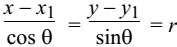# Parametric Form of Straight Line

If two variables, say x and y, are functions of a third variable, say θ, then the functions expressing x and y in terms of θ are called the parametric representations of x and y. The variable θ is called the parameter of the function.

Equation of a straight line passing through the point (x1, y1) and making an angle θ with x-axis.Any point on this line can be taken as (x1 + r cos θ, y+ r sin θ) where r is the algebraic distance.